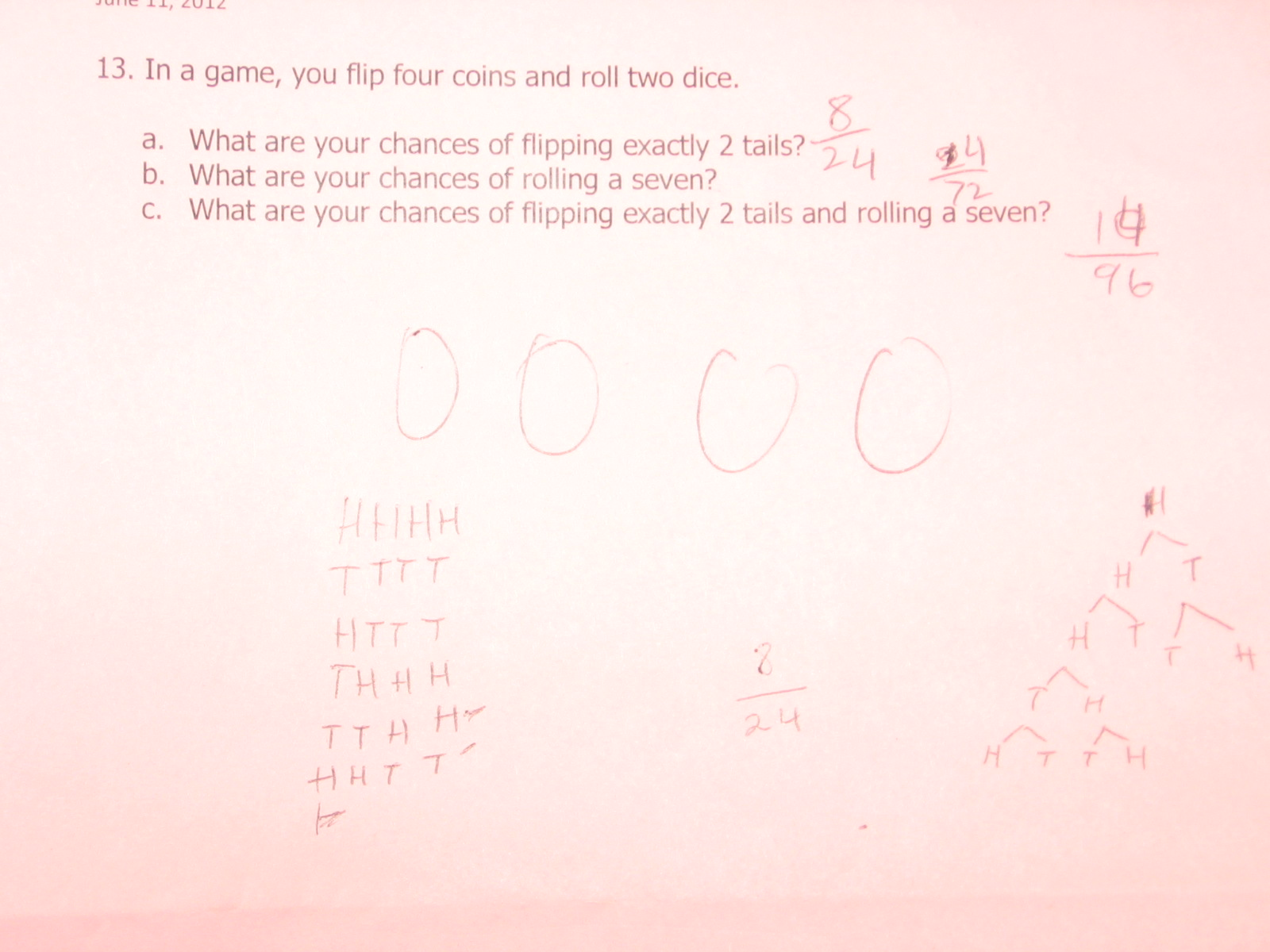Categories

# What are your chances of flipping exactly 2 tails?Can you explain where each of the student’s responses came from? As in, how did he arrive at 8/24, 14/96, and what is either 24/72 or 14/72.

## 2 replies on “What are your chances of flipping exactly 2 tails?”

I have no idea. My best guesses so far:

Four coins with two sides each makes 8. Also the tree diagram at the right has 8 endpoints (though I’m not quite sure what the algorithm was for deciding when to end; it was sometimes after 4 flips OR after reaching two tails, but other times it stopped after 2 flips even when there were only 0 or 1 tails).

There are 4 coins, and we want 2 tails, and 2*4 = 8.

The list of H’s and T’s on the left has six possibilities listed, of which two have two Ts (marked by the student), so that suggests that the 1/3 (8/24) answer is reasonable.

There are 2 sides per coin, 4 coins, thus by appending the digits we get 24. Or, more likely, there are 4 coins thus 4! = 24 ways to put them in order.

There are 2 dice, and we want to get a 7, hence 2 * 7 = 14. Each die has 6 sides, and there are 2 dice, so 2 * 6 * 6 = 72.

14 / (24 + 72) = 14/96.

I’m mostly pretty stumped, though — does anyone have some insight from the actual student in question?

Juliesays:

I agree with Josh for part a. I think the student was relying on their list. They made a list of six under 1 of the coins they drew. 2 of which had two tails. Therefore if they take the 6 possibilities and multiply it by four (thinking there are still 6 more lists to be made under each coin) they would get 24. Again, 2 of the possibilities met the requirement which means that 2×4 or 8 is how to get the numerator.

I agree with Josh’s assumptions for part b, especially without the presence of any work shown. However if the student made a case that based on part a they just need multiply the parts to find the numerator (This could be just grab and use a known operation too).

For part c, I think they kept the 14 because the chances of rolling a seven as they determined it was less likely than flipping two heads therefore that would be the one to use…

I wonder how many students answered 0 to part b and c. I would have. I have no chance to roll a ‘7’ assuming I have two six-sided die. Technically you should reword it to say “What is the probability that the sum of two die when rolled together would equal 7″…just the assessment geek in me.